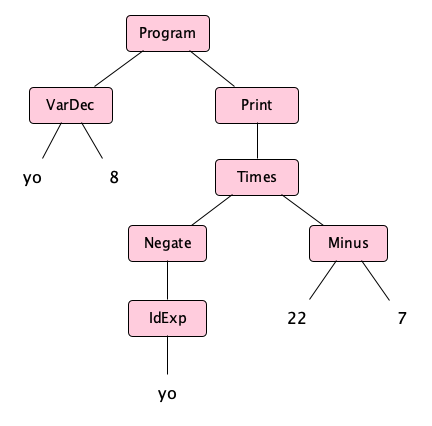# Programming Language Specification

How can we define a programming language?

## Let’s Design a Language

Let’s consider the language of infix integer arithmetic expressions with optional parentheses, the operators plus, minus, times, divide, and unary negation, and with spaces and tabs allowed between numbers and operators. We’ll call the language Ael, for Arithmetic Expression Language.

### Getting Started

When designing a language, it’s a good idea to start by sketching forms that you want to appear in your language as well as forms you do not want to appear.

ExamplesNon-Examples
432
24* (31/899  +3-0)/(54 /2+  4+2*3)
(2)
8*(((3-6)))

43 2
24*(31/// /)/(5+---+))

## Applying the Specification

Let’s determine the meaning of this string:

 -8     * (22-  7)


The character string is:

SPACE HYPHEN DIGIT EIGHT SPACE TAB ASTERISK SPACE LEFTPAREN DIGIT TWO DIGIT TWO HYPHEN SPACE SPACE DIGIT SEVEN RIGHTPAREN

Let’s apply the lexical rules to tokenize the string:

space - numlit(8) space space * space ( numlit(22) - space space numlit(7) )

The spaces will be skipped eventually, so we can just as well view the token stream like this:

- numlit(8) * ( numlit(22) - numlit(7) )

Parsing uncovers the derivation tree, also known as the concrete syntax tree:The static semantics is applied to the concrete syntax tree to produce the abstract syntax tree, namely: $$\{ \mathsf{Times} \; \{ \mathsf{Negate} \; \{\mathsf{Numlit}\;8\} \} \{ \mathsf{Minus} \; \{\mathsf{Numlit}\;22\} \; \{\mathsf{Numlit}\;7\} \} \}$$

which might be a little easier to read like this: $$\begin{array}{l} \{ \mathsf{Times} \\ \;\;\;\; \{ \mathsf{Negate} \; \{\mathsf{Numlit}\;8\} \} \\ \;\;\;\; \{ \mathsf{Minus} \; \{\mathsf{Numlit}\;22\} \; \{\mathsf{Numlit}\;7\} \} \} \end{array}$$

or even easier to read in tree form like this:which, informally can be shortened to:Notice how ASTs are way simpler than concrete trees. In fact in real compilers, unless the language is extraordinarily simple, you never see a concrete tree! Parsers generally go straight to the abstract syntax tree (though there are exceptions).

Finally, we apply the dynamic semantics to the AST to compute the meaning: $\begin{array}{l} \mathscr{E} \{\mathsf{Times}\;\{\mathsf{Negate}\;\{\mathsf{Numlit}\;8\}\}\{\mathsf{Minus}\;\{\mathsf{Numlit}\;22\}\;\{\mathsf{Numlit}\;7\}\}\} \\ \;\;\;\; = \mathscr{E} \{\mathsf{Negate}\;\{\mathsf{Numlit}\;8\}\} \times \mathscr{E}\{\mathsf{Minus}\;\{\mathsf{Numlit}\;22\}\;\{\mathsf{Numlit}\;7\}\}\} \\ \;\;\;\; = -\mathscr{E}\,\{\mathsf{Numlit}\;8\} \times (\mathscr{E}\{\mathsf{Numlit}\;22\} - \mathscr{E}\{\mathsf{Numlit}\;7\}) \\ \;\;\;\; = -8 \times (22-7) \\ \;\;\;\; = -8 \times 15 \\ \;\;\;\; = -120 \end{array}$

Exercise: Give the token stream and draw the concrete and abstract syntax trees for the Ael expression 98 * (247 - 6) / 3 / 100 - 701.
Exercise: See if you can find any high-level programming languages in which (* (- 8) (- 22 7)) is the actual way to write arithmetic expressions. Hint: several do exist. Why would anyone design a language this way?